# Solution assignment 10 Quadratic equations (factorizing)

### Assignment 10

Try to solve by factorization: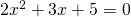### Solution

Because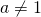and dividing both sides by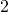yields fractions for the coefficients, factorization will not be applied. Applying the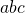-formula is the best way but will not give a solution because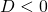(see also Quadratic equations (abc-formula)).

0